# SPSS tutorials

BASICS DATA ANALYSIS T-TEST ANOVA CHI-SQUARE TEST

# SPSS One-Way ANOVA with Post Hoc Tests Tutorial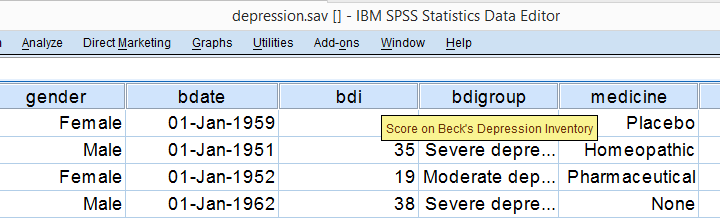A hospital wants to know how a homeopathic medicine for depression performs in comparison to alternatives. They adminstered 4 treatments to 100 patients for 2 weeks and then measured their depression levels. The data, part of which are shown above, are in depression.sav.

## Data Inspection - Split Histogram

Before running any statistical test, always make sure your data make sense in the first place. In this case, a split histogram basically tells the whole story in a single chart. We don't see many SPSS users run such charts but you'll see in a minute how incredibly useful it is. The screenshots below show how to create it.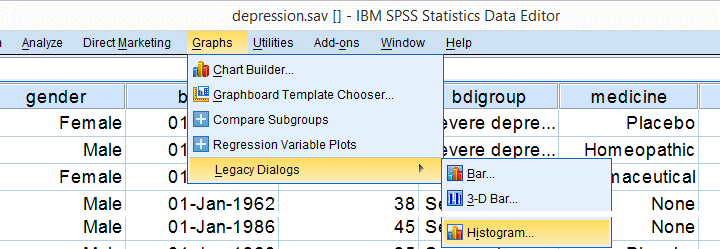In stepbelow, you can add a nice title to your chart. We settled for “Distribution BDI per Medicine”.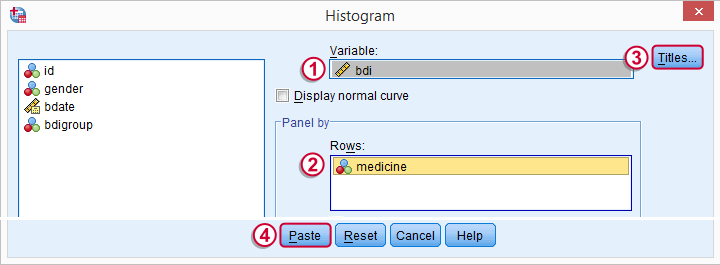## Syntax for Split Histogram

Clicking results in the syntax below. Running it creates our chart.

*Run histograms of BDI scores for the four medicines separately.

GRAPH
/HISTOGRAM=bdi
/PANEL ROWVAR=medicine ROWOP=CROSS
/TITLE='Distribution BDI per Medicine'.

## Result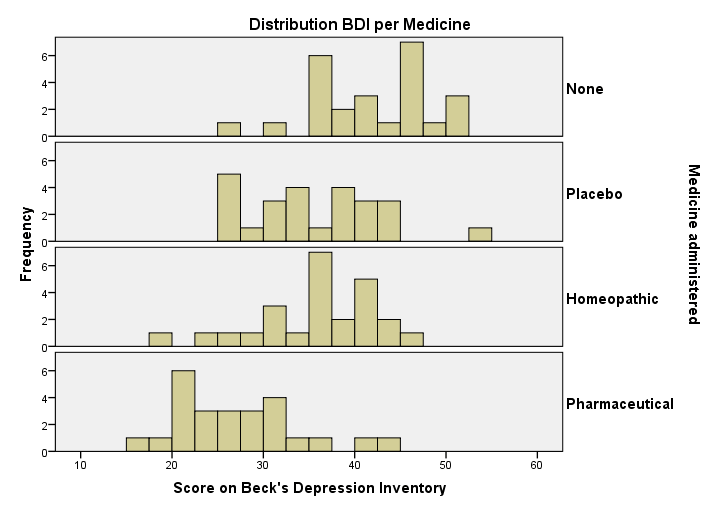These simple charts give a lot of insight into our data. The important points are:

• All distributions look plausible. We don't see very low or high BDI scores that should be set as user missing values and the BDI scores even look reasonably normally distributed.
• The medicine “None” results in the highest BDI scores, indicating the worst depressive symptoms. “Pharmaceutical” results in the lowest levels of depressive illness and the other two treatments are in between.
• The four histograms are roughly equally wide, suggesting BDI scores have roughly equal variances over our four medicines.

## Means Table

We'll now take a more precise look at our data by running a means table. We could do so from AnalyzeCompare MeansMeans but the syntax is so simple that just typing it is probably faster.

*Run basic means table.

means bdi by medicine
/cells count min max mean variance.

*Note: use /cells to choose which columns you'd like in which order.

## Result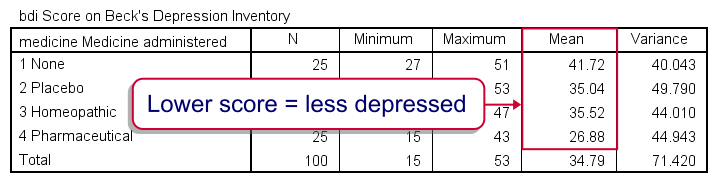Unsurprisingly, our table mostly confirms what we already saw in our histogram. Note (under “N”) that each medicine has 25 observations so these two variables don't contain any missing values.
So can we conclude that “Pharmaceutical” performs best and “None” performs worst? Well, for our sample we can. For our population (all people suffering from depression) we can't. The basic problem here is that samples differ from the populations from which they are drawn. If our four medicines perform equally well in our population, then we may still see some differences between our sample means. However, large sample differences are unlikely if all medicines perform equally in our population. For an outstanding explanation of this reasoning, read up on ANOVA - What Is It?
The question we'll now answer is: are the sample means different enough to reject the null hypothesis that the mean BDI scores in our populations are all equal?

## ANOVA - Omnibus Test and Post Hoc Tests

We often run ANOVA in 2 steps:

• we first test if all means are equal. This is often called the omnibus test. “Omnibus” is Latin for “about everything”.
• if we conclude that not all means are equal, we sometimes test precisely which means are not equal. This involves post hoc tests. “Post hoc” is Latin for “after that” in which “that” refers to the omnibus test. Right?

This standard procedure suggests that you should only run post hoc tests if the omnibus test
is “statistically significant”.
However, it could be argued that you should always run post hoc tests. In some fields like market research, this is pretty common. Reversely, you could argue that you should never use post hoc tests because the omnibus test suffices: some analysts claim that running post hoc tests is overanalyzing the data. Many social scientists are completely obsessed with statistical significance -because they don't understand what it really means- and neglect what's more interesting: effect sizes and confidence intervals.
In any case, the idea of post hoc tests is clarified best by just running them. But before doing so, let's take a quick look at the assumptions required for running ANOVA in the first place.

## ANOVA Assumptions

Our ANOVA will run fine in SPSS but we can take the results seriously only if our data satisfy 3 assumptions:

• Independent observations often holds if each case (row of cells in SPSS) represents a unique person or other statistical unit. That is, we usually don't want more than one row of data for one person, which holds for our data;
• Normally distributed variables in the population seems reasonable if we look at the histograms we inspected earlier. Besideds, violation of the normality assumption is no real issue for larger sample sizes due to the central limit theorem.
• Homogeneity means that the population variances of BDI in each medicine group are all equal, reflected in roughly equal sample variances. Again, our split histogram suggests this is the case but we'll try and confirm this by including Levene's test when running our ANOVA.

## Running our ANOVA in SPSS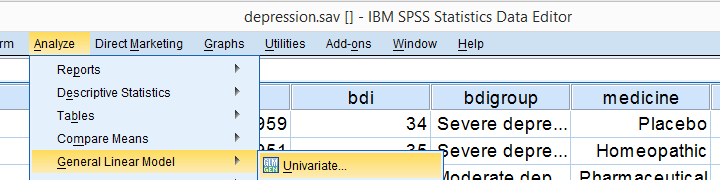There's many ways to run the exact same ANOVA in SPSS. Today, we'll go for General Linear Model because creates nicely detailed output.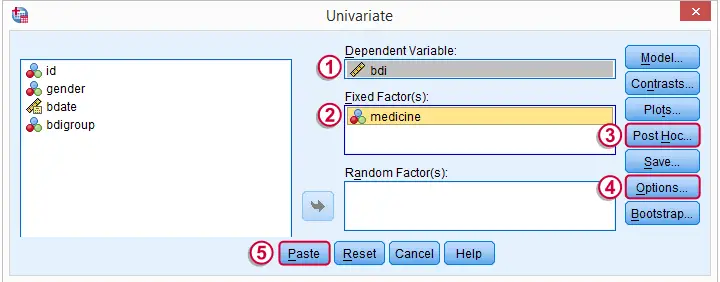We'll briefly jump into and before pasting our syntax.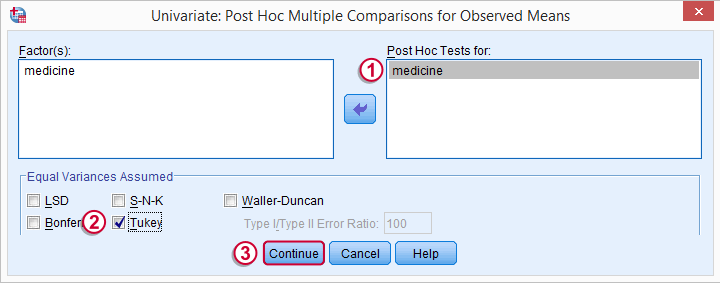The post hoc test we'll run is Tukey’s HSD (Honestly Significant Difference), denoted as “Tukey”. We'll explain how it works when we'll discuss the output.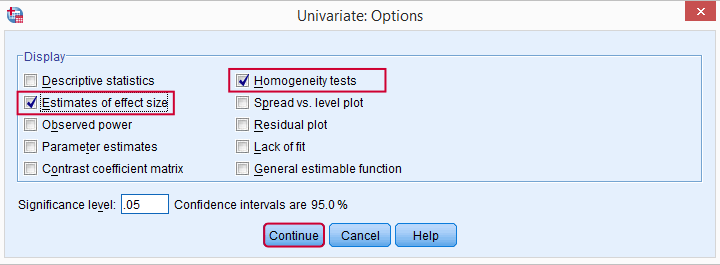“Estimates of effect size” refers to partial eta squared. “Homogeneity tests” includes Levene’s test for equal variances in our output.

## SPSS Post Hoc ANOVA Syntax

Following the previous screenshots results in the syntax below. We'll run it and explain the output.

*ANOVA syntax with Post Hoc (Tukey) test, homogeneity (Levene's test) and effect size (partial eta squared).

UNIANOVA bdi BY medicine
/METHOD=SSTYPE(3)
/INTERCEPT=INCLUDE
/POSTHOC=medicine(TUKEY)
/PRINT=ETASQ HOMOGENEITY
/CRITERIA=ALPHA(.05)
/DESIGN=medicine.

## SPSS ANOVA Output - Levene’s Test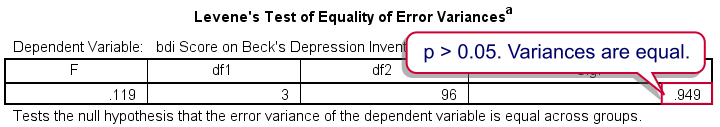Levene’s Test checks if the population variances of BDI for the four medicine groups are all equal, which is a requirement for ANOVA. As a rule of thumb, we reject the null hypothesis if p (or “Sig.”) < 0.05. In our case, p = 0.949 so we do not reject the null hypothesis of equal variances (or homogeneity). We assume the population variances are all equal so this ANOVA assumption is met by our data.

## SPSS ANOVA Output - Between Subjects Effects

The table below reports the aforementioned ANOVA omnibus test.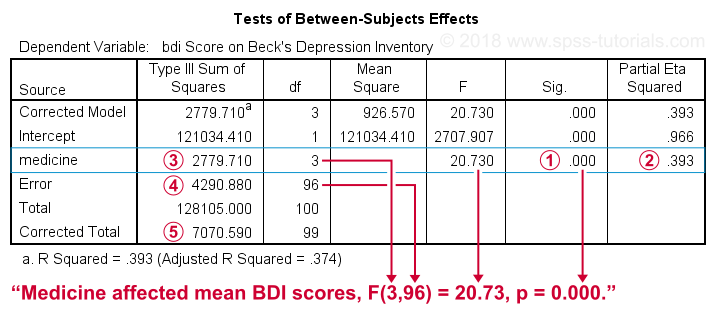Our null hypothesis is that the population means are equal for all medicines adminstered. P (“Sig.”) = 0.000 -way less than 0.05- so we reject this hypothesis: the population means are not all equal. Some medicines result in lower mean BDI scores than other medicines.The different medicines administered account for some 39% of the variance in the BDI scores. This is the effect size as indicated by partial eta squared.
Partial Eta Squared isthe Sums of Squares for medicine divided bythe corrected total sums of squares (2780 / 7071 = 0.39).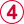Sums of Squares Error represents the variance in BDI scores not accounted for by medicine. Note that+=.

## SPSS ANOVA Output - Multiple Comparisons

So far, we only concluded that our four population means being all equal is very unlikely. So exactly which mean differs from which mean? Well, the histograms and means tables we ran before our ANOVA point us in the right direction. However, we'll try and back that up with a more formal test: Tukey’s HSD as shown in the multiple comparisons table.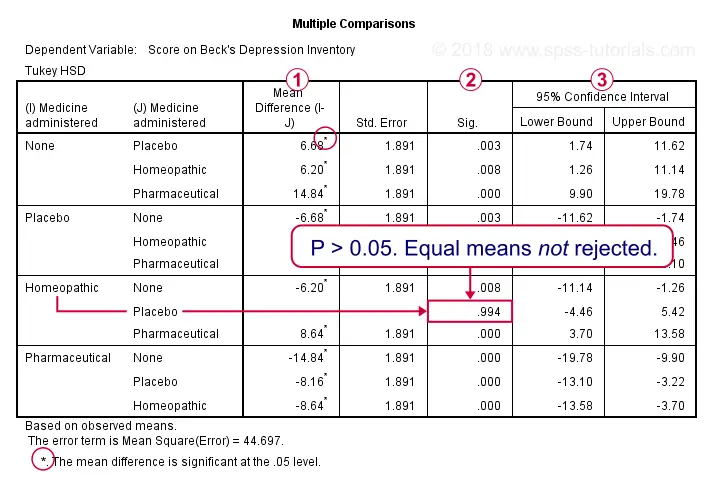Right, now comparing 4 means results in (4 - 1) x 4 x 0.5 = 6 distinct comparisons, each of which is listed twice in this table. There's three ways for telling which means are likely to be different:Statistically significant mean differences are flagged with an asterisk (*). For instance, the very first line tells us that “None” has a mean BDI score of 6.7 points higher than the placebo -which is quite a lot actually since BDI scores can range from 0 through 63.As a rule of thumb, “Sig.” < 0.05 indicates a statistically significant difference between two means.A confidence interval not including zero means that a zero difference between these means in the population is unlikely.
Obviously,,andresult in the same conclusions.

## SPSS ANOVA - APA Reporting Post Hoc Tests

So far, so good: we ran and interpreted an ANOVA with post hoc tests. However, the tables we created don't come even close to APA standards. We can run a much better table with the CTABLES syntax below. Honestly, I'm not sure how -or even if- it could be created from the menu but you can hopefully reuse it after just replacing the 2 variable names.

## SPSS CTABLES Syntax for APA ANOVA Table

*APA ANOVA table - means, SD's and Bonferroni corrected pairwise t-tests.

ctables
/table bdi [s] [count 'n' f3 mean 'Mean' f3.2 stddev 'SD' f3.2] by medicine [c]
/categories variables = medicine total = yes position = after
/slabels position = row
/titles title = 'Table 1 - Mean BDI Scores per Medicine with Bonferroni Adjusted Pairwise T-Tests'
/comparetest type = mean style = simple merge = yes.

*Note: running this requires SPSS custom tables license.

## Result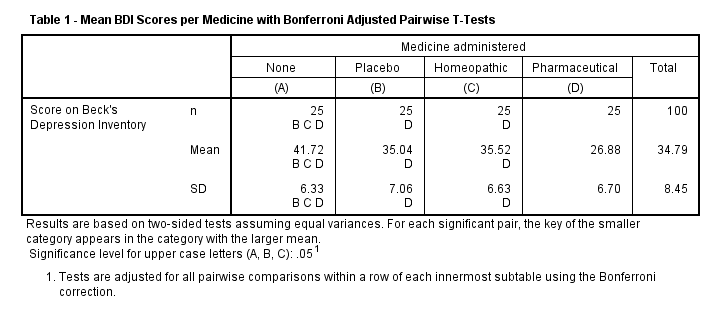First off, the capitals in this table (A, B and so on) indicate which means differ. SPSS also flags standard deviations and sample sizes. This is utter stupidity because these are not compared. They always have the same flags as the means. So just ignore everything except the actual means here.
Understanding this table starts with carefully reading its footnotes. First off, “two-sided tests” refers to independent samples t-tests using 2-tailed significance.
Next, each statistically significant difference is indicated only once in this table. As indicated before, 4 means yield 6 unique pairs of means. Altogether, the table has 5 significance markers (A, B and so on). This means that only (6 - 5 =) only 1 pair of means do not differ. After some puzzling these turn out to be homeopathic versus placebo. This is the exact same conclusion we drew earlier from our pairwise comparisons (Tukey’s) table.

So that's it for now. I hope this tutorial helps you to run ANOVA with post hoc tests confidently. If you have any suggestions, please let me know by leaving a comment below.

# Let me know what you think!

*Required field. Your comment will show up after approval from a moderator.

# This tutorial has 33 comments

•### By abdo nazim on March 4th, 2018

how to compare multible means using superscripts after one way ANOVA and post hoc LSD?

•### By Veronica Ocampo on March 25th, 2017

Thank you! A very down-to-earth tutorial. The screenshots are invaluable plus the ease in interpretation of all the numbers. A great help in completing my homework!

•### By Andrea on February 10th, 2017

Hello, I wanted to know if ANOVA works out when it comes to prove independence between variables. Particularly, I am studying how several conditions regarding an industrial process (such as temperatures and flows) affect one of the chemical components of the product. I know that the dependent variable would be the amount of the chemical component and that the process conditions would be the fixed factors. But how can I interpret the interaction between this variables (and the combination of them) with the chemical component with the ANOVA results?

•### By Ruben Geert van den Berg on October 28th, 2016

Hi Ernest, thanks for the compliment and a great question!

If the sample sizes are (roughly) equal, don't bother about homogeneity being violated because the F-test is pretty robust in this case. However, if you have sharply unequal sample sizes, run a Kruskal-Wallis test besides the ANOVA since it doesn't require equal variances.

For larger samples, both tests will often lead to the same conclusion so the KW-test kinda backs up your ANOVA conclusion. However, if they lead to different conclusions, the KW-test conclusion should probably prevail.

Hope that helps!

•### By Ernest on October 28th, 2016

Beautiful explanation. So what do i do if my Levene's Test gives a sig of 0.00? Does it mean there are unequal variances, and what do i do?